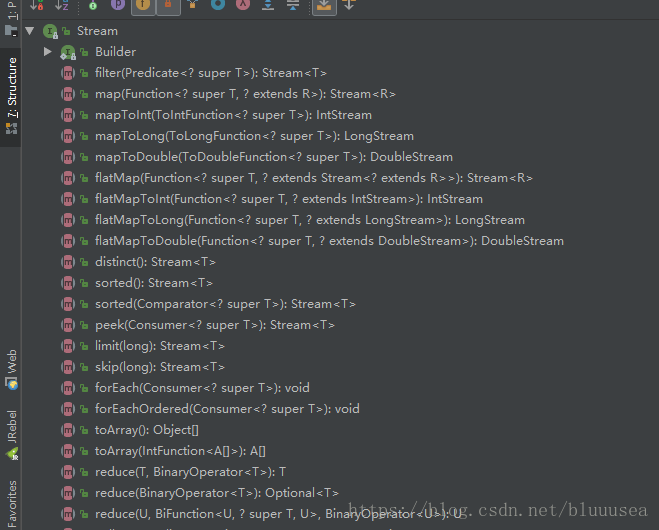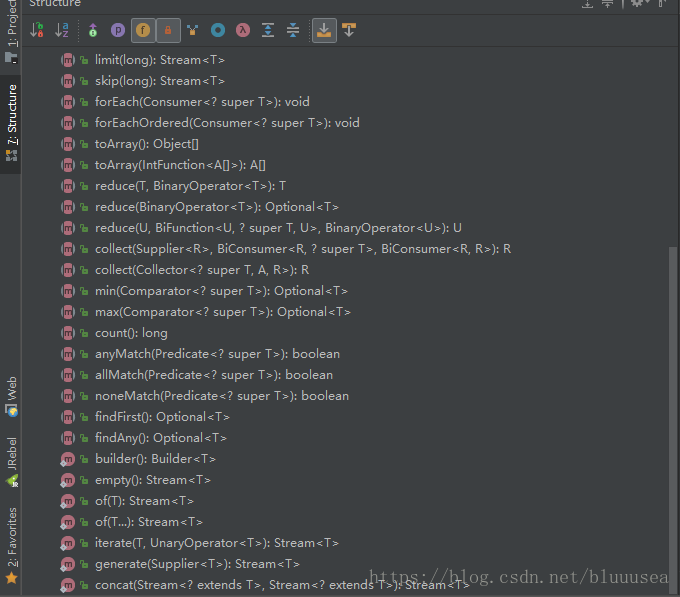• 如果您觉得本站非常有看点，那么赶紧使用Ctrl+D 收藏吧

# JAVA8新特性–集合流操作Stream

Stream类全路径为：java.util.stream.Stream

Java 8 中的 Stream 是对集合（Collection）对象功能的增强，它专注于对集合对象进行各种非常便利、高效的聚合操作（aggregate operation），或者大批量数据操作 (bulk data operation)。Stream API 借助于同样新出现的 Lambda 表达式，极大的提高编程效率和程序可读性。

• stream() − 为集合创建串行流。
• parallelStream() − 为集合创建并行流。

Stream的的中间操作（intermediate）和最终操作（terminal）都包含哪些方法可以从类结构中看到：• 中间操作（intermediate）主要有以下方法（此类型的方法返回的都是Stream对象）：
map (mapToInt, flatMap 等)、 filter、 distinct、 sorted、 peek、 limit、 skip、 parallel、 sequential、 unordered

• 终端操作（terminal）主要有以下方法：
forEach、 forEachOrdered、 toArray、 reduce、 collect、 min、 max、 count、 anyMatch、 allMatch、 noneMatch、 findFirst、 findAny、 iterator

• filter：通过设置条件来过滤元素。
``````List<String> list = Arrays.asList("aaa","ddd","bbb","ccc","a2a","d2d","b2b","c2c","a3a","d3d","b3b","c3c");

list.stream()
.filter((s)->s.contains("a"))
.forEach(s -> System.out.println(s));
``````

aaa
a2a
a3a

• map：就是将对应的元素使用给定方法进行转换。
``````list.stream()
.filter((s)->s.contains("a"))
.map((s)-> s + "---map")
.forEach(s -> System.out.println(s));

``````

aaa—map
a2a—map
a3a—map

• mapToInt：和map方法进行一样的操作，但是转换函数必须返回int类型
• mapToLong：和map方法进行一样的操作，但是转换函数必须返回long类型
• mapToDouble：和map方法进行一样的操作，但是转换函数必须返回double类型
此处以mapToInt进行演示：
``````list.stream()
.filter((s)->s.contains("a"))
.mapToInt((s)-> s.hashCode())
.forEach(s -> System.out.println(s));
``````

96321
94864
94895

• flatMap：如果流的元素为数组或者Collection，flatMap就是将每个Object[]元素或Collection<Object>元素都转换为Object元素，如下：

Stream<String[]> 转换为 Stream<String>
Stream<Set> 转换为 Stream<String>
Stream<List> 转换为 Stream<String>
Stream<List> 转换为 Stream<Object>

``````List<String[]> setList =  new ArrayList<>();
//使用map方法
setList.stream()
.map(s->Arrays.stream(s))
.forEach(s-> System.out.println("map==" + s));
//使用flatMap方法
setList.stream()
.flatMap(s->Arrays.stream(s))
.forEach(s-> System.out.println("flatMap==" + s));
``````

flatMapaa
flatMapbb
flatMapcc
flatMapdd
flatMapee
flatMap==ff

• distinct：将集合中的元素去重。
``````List<String> disList = Arrays.asList("aaa","ddd","bbb","ddd","aaa");
disList.stream()
.distinct()
.forEach(s-> System.out.println(s));
``````

aaa
ddd
bbb

• sorted：将集合中的元素排序。
``````List<Integer> integerList = Arrays.asList(1,2,3,4);
integerList.stream()
.sorted()
.forEach(s-> System.out.println(s));
``````

1
2
3
4

``````integerList.stream()
.sorted((s1,s2)->s2.compareTo(s1))
.forEach(s-> System.out.println(s));
``````

4
3
2
1

• peek：生成一个包含原Stream的所有元素的新Stream，同时会提供一个消费函数即引用的方法A，当Stream每个元素被消费的时候都会先执行新Stream给定的方法A。peek是中间操作，如果pee后没有最终操作，则peek不会执行。
``````integerList.stream()
.peek(s-> System.out.println("peek = "+s));
``````

``````integerList.stream()
.peek(s-> System.out.println("peek = "+s))
.forEach(s-> System.out.println("forEach = "+s));
``````

peek = 1
forEach = 1
peek = 2
forEach = 2
peek = 3
forEach = 3
peek = 4
forEach = 4

• limit：返回Stream的前n个元素。
``````integerList.stream()
.limit(1)
.forEach(s-> System.out.println(s));
``````

1

• skip：删除Stream的前n个元素。
``````integerList.stream()
.skip(1)
.forEach(s-> System.out.println(s));
``````

2
3
4

• forEach：遍历Stream中的每个元素，前面每个例子都有使用，此处不再演示。
• forEachOrdered:遍历Stream中的每个元素。
区别
在串行流（stream）中没有区别，在并行流（parallelStream）中如果数据源是有序集合，forEachOrdered输出顺序与数据源中顺序一致，forEach则是乱序。

``````integerList.parallelStream()
.forEach(s-> System.out.println(s));
``````

3
1
4
2

``````integerList.parallelStream()
.forEachOrdered(s-> System.out.println(s));
``````

2
1
3
4

• toArray：将流转换为Object[]或者指定类型的数组。
``````Object[] array = integerList.stream().toArray();
String[] strArr = integerList.stream().toArray(String[]::new);
``````
• reduce：将集合中的每个元素聚合成一条数据。有三种情况：
• reduce(BinaryOperator accumulator)：此处需要一个参数，返回Optional对象：
``````Optional<Integer> reduce = integerList.stream().reduce((a, b) -> a + b);
``````
• reduce(T identity, BinaryOperator accumulator)：此处需要两个参数，第一个参数为起始值，第二个参数为引用的方法。从起始值开始，每个元素执行一次引用的方法（方法引用的中的两个参数：第一个参数为上个元素执行方法引用的结果，第二个参数为当前元素）。
``````int integer = integerList.stream().reduce(5,(a, b) -> a + b);
System.out.println(integer);
``````

15

• reduce(U identity, BiFunction<U, ? super T, U> accumulator, BinaryOperator<U> combiner)：此处需要三个参数。此方法用在并发流（parallelStream）中，启动多个子线程使用accumulator进行并行计算，最终使用combiner对子线程结果进行合并，返回identity类型的数据，看到有篇文章对这个解释比较清楚：java8中3个参数的reduce方法怎么理解？
• collect：将流转换成集合或聚合元素。有两种情况。接受一个参数和接受三个参数（三个参数在并发流parallelStream中使用），此处介绍一个参数的情况，单个参数接受的参数类型为Collector，Collectors 类实现了很多归约操作，详见：java8之collector
``````List<Integer> collects = integerList.stream()
.filter(a -> a > 1)
.collect(Collectors.toList());
System.out.println(collects);
``````

[2, 3, 4]

• min:获取集合中最小值。
``````Integer min = integerList.stream()
.filter(a -> a > 1)
.min((Integer a, Integer b) -> a.compareTo(b))
.get();
System.out.println(min);
``````

2

• max：获取集合中最大值。
``````Integer max = integerList.stream()
.filter(a -> a > 1)
.max((Integer a, Integer b) -> a.compareTo(b))
.get();
System.out.println(max);
``````

4

• count：获取集合中元素个数
``````long count = integerList.stream()
.filter(a -> a > 1)
.count();
System.out.println(count);
``````

3

• anyMatch： Stream 中任意一个元素符合传入的 predicate，返回 true
• allMatch：Stream 中全部元素符合传入的 predicate，返回 true
• noneMatch：Stream 中没有一个元素符合传入的 predicate，返回 true
``````boolean b = integerList.stream()
.anyMatch(s -> s > 0);
boolean b1 = integerList.stream()
.allMatch(s -> s > 0);
boolean b2 = integerList.stream()
.noneMatch(s -> s > 0);
System.out.println("anyMatch = " + b);
System.out.println("allMatch = " + b1);
System.out.println("noneMatch = " + b2);
``````

anyMatch = true
allMatch = true
noneMatch = false

• findFirst：如果数据源是有序集合，返回Stream 中第一个元素的Optional对象，如果是无序集合，则返回Stream 中任意一个元素的Optional对象。
``````Integer first = integerList.stream()
.findFirst()
.get();
System.out.println(first);
``````

2

• findAny：返回Stream 中任意一个元素的Optional对象。
``````Integer any = integerList.stream()
.findAny()
.get();
System.out.println(any);
``````

2

Stream还有几个静态方法，返回都是Stream对象。

builder：返回一个Builder对象，Builder对象在调用build()返回Stream对象。
empty：返回一个空的有序的Stream对象。
of：返回包含单个元素的有序的Stream对象。
iterate：返回一个无限元素的有序的Stream对象。需要两个参数，第一个参数为初始值，第二个参数为要引用的方法，然后会通过递归循环调用引用的方法。

``````Stream.iterate(2,s->s+s)
.limit(10)
.forEach(s-> System.out.println(s));
``````

2
4
8
16
32
64
128
256
512
1024

generate：返回一个无限元素的无序的的Stream对象。需要一个参数，参数为引用的方法，然后会通过循环调用引用的方法来生成元素，常用于生成常量Stream和随机元素Stream。
concat：将两个Stream连接成一个Stream。需要两个Stream作为参数，如果两个Stream都是有序的并且无论参数Stream是否是并行Stream，得到的都是有序的Stream。输出元素顺序为先输出第一个Stream的元素，然后输出第二个Stream的元素。当结果Stream关闭时候，两个参数Stream同时关闭。

``````Stream.concat(integerList.stream(),disList.stream())
.forEach(s-> System.out.println(s));
``````

2
1
3
4
11
aaa
ddd
33
bbb
ddd
aaa